# Between Curves Tools

## Overview

This program uses numeric integration to find information about the area between two curves. It also does a very nice job of adjusting the bounds of the graph window so that you get a visual representation of what is happening. The program can find the area between two curves,the volume of rotation generated by revolving that area around any vertical or horizontal line, and the centroid of a region.

## Instructions

### Area Between Curves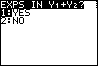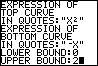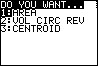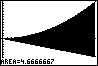Either enter the upper and lower expressions in Y1 and Y2 (and select that the exps are already in Y1 + Y2) or select that the exps are not in Y1 + Y2 and enter them as prompted (as in this example.) Then enter the lower and upper bounds for the integration. Select AREA from the menu, and watch it go. This example found the area between the curves Y=X^2 and Y=-X from 0 to 2. Notice that the graph is drawn to take up the entire screen of the calculator.

### Volume of Circular Revolution

#### Around a Horizontal Line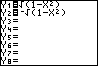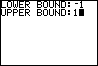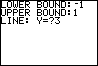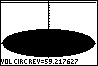This example rotates the circle of radius 1 around the origin around the vertical line Y=3. If you viewed the three dimensional image it would look like a donut or an intertube standing vertically. Enter the expressions and the bound just as you would for finding area. In this example the expressions were put in Y1 and Y2 before the program was run. Select Volume of Circular Revolution and then Horizontal line from the menu's. Put the equation for the line (ie. a number not in quotes). This example uses 3. Press enter and it will draw the thing for you. Notice that it puts emphasis on fitting the figure and the axis of rotation onto the screen. therefore the circle doesn't look very circular.

#### Around a Vertical Line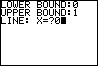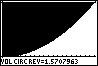This example makes a 3-D object that is shaped like a cereal bowl that is filled solid. The expressions are y1=1 andY2=X^2. The instructions are almost identical to that of the rotation around the horizontal line except that vertical line should be selected.

### Centroid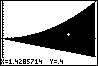Finding the centroid of a region is very similar to finding the area of the region. This example uses the same figure as the example for finding area. However, instead of selecting 'AREA' from the menu, select 'CENTROID'. The centroid point will be drawn and the X, Y coordinates of the centroid will be displayed.

### Bounding Errors

• Problem: I keep having to re-enter my upper and lower bounds!

The lower bound must be less than the upper bound.

• Problem: I get the message 'Y1 IS NOT ALWAYS > Y2'

The expression in Y1 must evaluate to be greater than or equal to Y2 at every point in the bound. If it isn't (ie. the lines graphs cross some where) integration will not give you the area and this program will not be able to handle it. (Integration will try to subtract the areas where the bottom equation is above the top one.) When this happens, break the bound up into two or more sections so that the graphs don't cross in each section. Find the area of each section and add them all together. Sometimes the graphs cross at the edge of the bound but the calculator doesn't have enought significant figures to know this. If you get an error message when you know the graphs don't cross but they just touch at the edges of the bound, try making the bound a tiny bit smaller. For example instead of entering 0, 2 as the bound, enter .0000000001, 1.999999999.